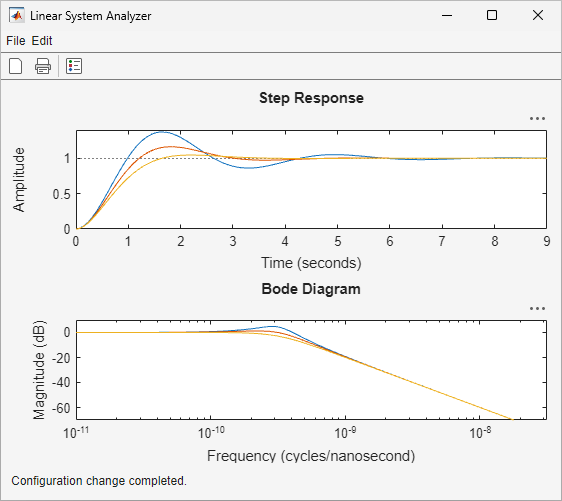Documentation

### This is machine translation

Mouseover text to see original. Click the button below to return to the English version of the page.

# Linear System Analyzer

Analyze time and frequency responses of linear time-invariant (LTI) systems

## Description

The Linear System Analyzer app lets you analyze time and frequency responses of LTI systems. Using this app, you can:

• View and compare the response plots of SISO and MIMO systems, or of several linear models at the same time.

• Generate time response plots such as step, impulse, and time response to arbitrary inputs.

• Generate frequency response plots such as Bode, Nyquist, Nichols, singular-value, and pole-zero plots.

• Inspect key response characteristics, such as rise time, maximum overshoot, and stability margins.

### Available Plots

Linear System Analyzer can generate the following response plots:

• Step response

• Impulse response

• Simulated time response to specified input signal

• Simulated time response from specified initial conditions (state-space models only)

• Bode diagram (magnitude and phase, or magnitude alone)

• Nyquist plot

• Nichols plot

• Singular value plot

• Pole/zero map and I/O pole/zero map## Open the Linear System Analyzer App

• MATLAB® Toolstrip: On the Apps tab, under Control System Design and Analysis, click the app icon.

• MATLAB command prompt: Enter `linearSystemAnalyzer`.

## Programmatic Use

expand all

`linearSystemAnalyzer` opens the Linear System Analyzer app with no LTI systems to analyze. To specify a system to analyze, select File > Import.

`linearSystemAnalyzer(sys1,sys2,...,sysn)` opens Linear System Analyzer and displays the step response of one or more dynamic system models, `sys1`, `sys2`, ..., `sysn`. Such models include:

• Numeric LTI models such as `tf`, `zpk`, or `ss` models.

• Identified models such as `idtf`, `idss`, or `idproc` (requires System Identification Toolbox™ software).

• Generalized LTI models such as `genss` or `uss` models. For generalized LTI models without uncertainty, Linear System Analyzer plots the response of the nominal value of the model. For generalized models with uncertainty, the app plots the responses of 20 random samples of the uncertain system. (Uncertain models require Robust Control Toolbox™ software.)

`linearSystemAnalyzer(sys1,LineSpec1,sys2,LineSpec2...,sysn,LineSpecn)` specifies the line style, marker, and color of each response plot. Specify plot styles using one, two, or three characters. For example, the following code uses red asterisks for the response of `sys1`, and a magenta dotted line for the response of `sys2`.

```linearSystemAnalyzer(sys1,'r-*',sys2,'m--'); ```

For more information about configuring this argument, see the `LineSpec` input argument of the `plot` function.

`linearSystemAnalyzer(plottype,___)` opens Linear System Analyzer and displays the response types specified by `plottype`. You can use this syntax with any of the previous input argument combinations. The `plottype` argument can be any one of the following:

• `'step'` — Step response.

• `'impulse'` — Impulse response.

• `'lsim'` — Linear simulation plot. When you use this plot type, the Linear Simulation Tool dialog box prompts you to specify an input signal for the simulation.

• `'initial'` — Initial condition plot (state-space models only). You can use the `extras` argument to specify the initial state. If you do not, the Linear Simulation Tool dialog box opens and prompts you to specify an initial state for the simulation.

• `'bode'` — Bode diagram.

• `'bodemag'` — Bode magnitude diagram.

• `'nyquist'` — Nyquist plot.

• `'nichols'` — Nichols plot.

• `'sigma'` — Singular value plot. (See `sigma`).

• `'pzmap'` — Pole/zero map.

• `'iopzmap'` — Pole/zero map of each input/output pair of the LTI system.

To open Linear System Analyzer with multiple response plots, use a cell array of up to six of these plot types for the `plottype` input argument. For example, the following command opens the app with a step response plot and a Nyquist plot for the system `sys`.

```linearSystemAnalyzer({'step';'nyquist'},sys) ```

`linearSystemAnalyzer(plottype,sys1,sys2,...,sysn,extras)` specifies additional input arguments specific to the type of response plot. `extras` can be one or more of the input arguments available for the function corresponding to the plot type. For example, suppose `plottype` is `'step'`. Then, `extras` enables you to use the additional arguments that you could use with the `step` command, such as the desired final time, `Tfinal`. Thus, the following command opens the app with a step response plot of `sys`, with a final time of `Tfinal`.

```linearSystemAnalyzer('step',sys,Tfinal) ```

If `plottype` is `'initial'`, you can use `extras` to supply the initial conditions `x0`, and other arguments such as `Tfinal`. For example:

```linearSystemAnalyzer('initial',sys,x0,Tfinal) ```

To determine appropriate arguments for `extras`, see the reference pages of the functions corresponding to each plot type, such as `step`, `bode`, or `initial`.

`h = linearSystemAnalyzer(___)` returns a handle to the Linear System Analyzer figure. You can use this syntax with any of the previous combinations of input arguments. Use the handle to modify previously opened Linear System Analyzer instances, as described in the next two syntaxes.

`linearSystemAnalyzer('clear',h)` clears the plots and data from the Linear System Analyzer corresponding to handle `h`. To clear multiple app instances at once, set `h` to a vector of handles.

`linearSystemAnalyzer('current',sys1,sys2,...,sysn,h)` adds the responses of the systems `sys1`, `sys2`, ..., `sysn` to the Linear System Analyzer corresponding to handle `h`. To update multiple app instances at once, set `h` to a vector of handles. If the new systems have different I/O dimensions from the currently displayed systems, the app clears the existing responses and displays only the new ones.